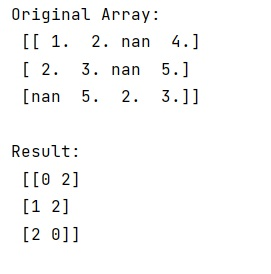# How to get the indices list of all NaN value in NumPy array?

Learn, how to get the indices list of all NaN value in NumPy array?
Submitted by Pranit Sharma, on January 07, 2023

NumPy is an abbreviated form of Numerical Python. It is used for different types of scientific operations in python. Numpy is a vast library in python which is used for almost every kind of scientific or mathematical operation. It is itself an array which is a collection of various methods and functions for processing the arrays.

## Problem statement

While creating a DataFrame or importing a CSV file, there could be some NaN values in the cells. NaN values mean "Not a Number" which generally means that there are some missing values in the cell.

Suppose we are given a NumPy array that contains some nan values and we need to get a list containing the indices of all the nan values in sequence.

## Finding the indices list of all NaN value in NumPy array

To find the indices list of all NaN value, we will use numpy.isnan() combined with numpy.argwhere.isnan() method produces a bool array indicating where the NaN values are. Hence, it returns the nan value and numpy.argwhere() returns the position of the nan values returned by numpy.isnan().

Let us understand with the help of an example,

## Python program to get the indices list of all NaN value in NumPy array

```# Import numpy
import numpy as np

# Creating an array
arr = np.array([[1,2,np.nan,4],[2,3,np.nan,5],[np.nan,5,2,3]])

# Display original array
print("Original Array:\n",arr,"\n")

# Getting a list of indices of nan values
res = np.argwhere(np.isnan(arr))

# Display result
print("Result:\n",res,"\n")
```

## Output

The output of the above program is: